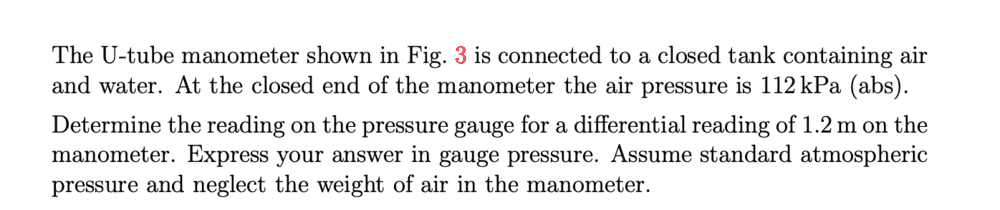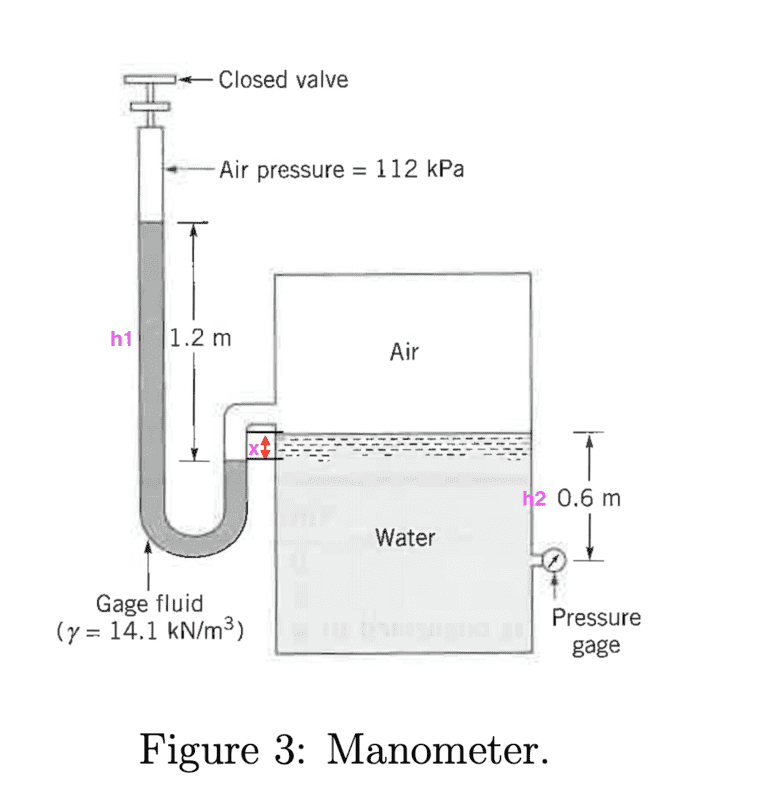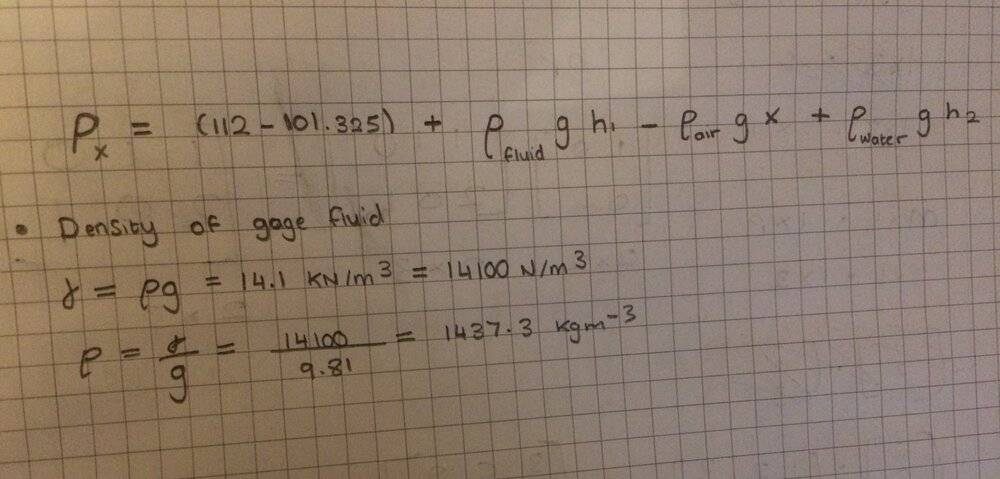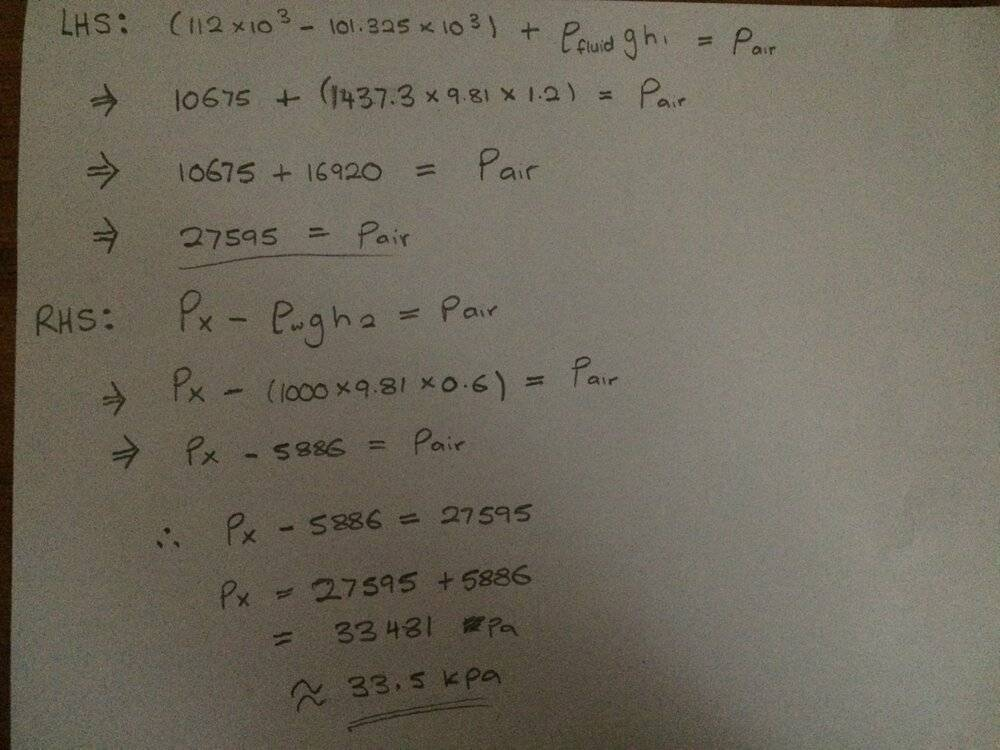# Calculating this gauge pressure reading

Bolter
Homework Statement:
See below
Relevant Equations:
pressure = rho x g x h
specific weight = rho x g
Hi everyone!
How do I go about solving this problem?I tried working out the gauge pressure using this but I have a few unknowns which won't make this possible such as what is the length of x which I labelled in the figureAny help would be appreciated! Thanks

Homework Helper
Gold Member
The U-tube manometer is telling you the pressure of the volume of air trapped in the tank.
The pressure gauge should be telling you the static pressure at that height of liquid, which surface is being pressured down by the trapped air.

Bolter
The U-tube manometer is telling you the pressure of the volume of air trapped in the tank.
The pressure gauge should be telling you the static pressure at that height of liquid, which surface is being pressured down by the trapped air.

Ok I get that, but I am not entirely sure how I can form an equation with that statement. Do I begin off by setting up an expression on the left and right side of the figure then equate them?

Homework Helper
Gold Member
The trapped pocket of air in the tank is simultaneously in contact with the surface of both liquids, having a unique pressure.
I would establish relations for each side around that common pressure.

I believe that height x is irrelevant, do you agree?

Last edited:
•Bolter
Bolter
The trapped pocket of air in the tank is simultaneously in contact with the surface of both liquids, having a unique pressure.
I would establish relations for each side around that common pressure.

I believe that height x is irrelevant, do you agree?

Thanks I got the right answer from making relations on both sides and then equalling those 2. Gauge pressure turned out to be 33.5 kPa•Lnewqban
Homework Helper
Gold Member
You are welcome.•